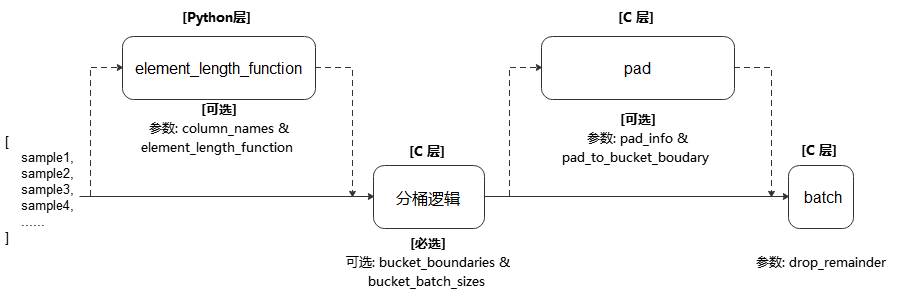# mindspore.dataset.Dataset.bucket_batch_by_length• column_names (list[str]) - 传递给参数 element_length_function 的数据列，用于计算数据的长度。

• bucket_boundaries (list[int]) - 指定各个分桶的上边界值，列表的数值必须严格递增。 如果有n个边界，则会创建n+1个桶，分配后桶的边界如下：[0, bucket_boundaries)，[bucket_boundaries[i], bucket_boundaries[i+1])，[bucket_boundaries[n-1], inf)，其中，0<i<n-1。

• bucket_batch_sizes (list[int]) - 指定每个分桶的批数据大小，必须包含 len(bucket_boundaries)+1 个元素。

• element_length_function (Callable, 可选) - 长度计算函数。要求接收 len(column_names) 个输入参数，并返回一个整数代表该条数据的长度。 如果未指定该参数，则参数 column_names 的长度必须为1，此时该列数据的shape值将被当做数据长度。默认值：None，不指定。

• drop_remainder (bool, 可选) - 当每个分桶中的最后一个批处理数据数据条目小于 bucket_batch_sizes 时，是否丢弃该批处理数据。默认值：False，不丢弃。

Dataset，按长度进行分桶和批处理操作后的数据集对象。

```>>> # Create a dataset where certain counts rows are combined into a batch
>>> # and drops the last incomplete batch if there is one.
>>> import numpy as np
>>> def generate_2_columns(n):
...     for i in range(n):
...         yield (np.array([i]), np.array([j for j in range(i + 1)]))
>>>
>>> column_names = ["col1", "col2"]
>>> dataset = ds.GeneratorDataset(generate_2_columns(8), column_names)
>>> bucket_boundaries = [5, 10]
>>> bucket_batch_sizes = [2, 1, 1]
>>> element_length_function = (lambda col1, col2: max(len(col1), len(col2)))
>>> # Will pad col2 to shape [bucket_boundaries[i]] where i is the
>>> # index of the bucket that is currently being batched.
>>> pad_info = {"col2": ([None], -1)}